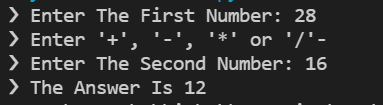How to Make a Simple Calculator Using Python

Published at - Dec 08, 2021

Here’s a small project for you if you’re new to Python. To develop your abilities, make a very basic calculator! I’ll teach you how to create one in this article.

Creating Inputs

We’ll need to make three inputs for the user to fill up with their chosen values.

We begin by creating num1, which is the first number the user must compute.

num1 = int(input("❯ Enter The First Number: "))

Second, we design an operator that the user may use to input data. If the user needs to multiply, for example, they may just type *.

operator = (input("❯ Enter '+', '-', '*' or '/'"))

Finally, we generate num2, which is the second number the user must compute.

num2 = int(input("❯ Enter The Second Number: "))

Remember to include int(integer) before the inputs!

Logic Writing

Now we’ll write the code for the calculator’s logic.

if Statements

We’ll use ifstatements to write code that adds, subtracts, multiplies, or divides using the calculator.

if operator == '+':

Subtraction

if operator == '-':

Multiplication

if operator == '*':

Division

if operator == '/':

That concludes the logic!

The Last Step

The only thing left to do is print it!

Make sure str(string) is present once again!

That’s all there is to it; the calculator is now complete!

Congratulations on this project, and good luck with your studies!

Here’s how it turned out:Source

I hope you enjoyed reading this lesson; you can follow me to read more tutorials from me in the future. Thank you for your time.

By becoming a Medium member, you can support me and your other favorite authors. Thanks! 👇 Join Medium with my referral link - Harendra Verma As a Medium member, a portion of your membership fee goes to writers you read, and you get full access to every story…medium.com